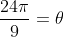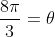Trigonometry : Angular Velocity

Example Questions

Example Question #1 : Angular Velocity

What is the formula for angular velocity?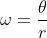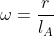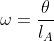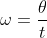Explanation:

Angular velocity is a measure of the time it takes to travel over a certain arc that is formed by a central angle.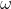is usually used to represent the angular velocity,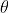is the measure of the central angle, andis the time it takes to travel from point A to B.  This formula looks similar to the measure of an arc,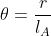, but we are considering the velocity in terms of the measure of the angle over the amount of time it took to cover that distance.Example Question #1 : Angle Applications

What is the angular velocity of an angle whose measure is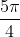when it took a point 6 seconds to rotate through the angle.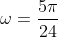radians/seconds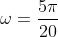radians/seconds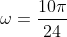radians/seconds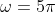radians/secondsradians/seconds

Explanation:

We have enough information to plug into the angular velocity formula to solve this problem.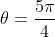and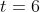seconds.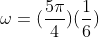So our answer isradians/seconds

Example Question #1 : Angle Applications

True or False: The angular velocity is a measure of an angle of rotation over time.

False

True

True

Explanation:

consider the figure below.  Think of line A being connected to a hinge whereis.  We are measuring the amount of time it takes for line A to rotate and align with line B.  This is a measure of angle rotation over time.Example Question #4 : Angular Velocity

What is the angular velocity of an angle whose measure is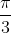when it took a point 10 seconds to rotate through the angle.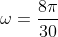radians/seconds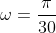radians/seconds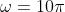radians/seconds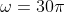radians/secondsradians/seconds

Explanation:

We have enough information to plug into the angular velocity formula to solve this problem.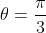and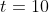seconds.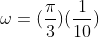And so our answer isradians/seconds.

Example Question #5 : Angular Velocity

If you are walking around a circular track and your angular velocity through the angle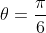is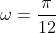, how long would it take you to complete a full rotation?

24 seconds

2 seconds

12 seconds

22 seconds

24 seconds

Explanation:

This is simply solving for an unknown.  Here our unknown is time,.  So we know that it takes you have an angular velocity ofthrough the angle.  Assuming that your angular velocity is consistent, we will be able to solve for.  First we must manipulate our original angular velocity formula to solve for.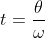Now we will plug in our known value forand the angle of a full rotation.  The angle of a full rotation is simply that of a full circle,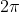.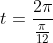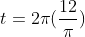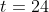So it takes you 24 seconds to complete a full rotation

Example Question #6 : Angular Velocity

You are on a carousel and have completed one full rotation in 15 seconds.  What is your angular velocity?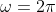radians/seconds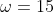radians/seconds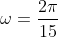radians/seconds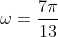radians/secondsradians/seconds

Explanation:

Even though we are not directly given an angle measurement, we are told the time it takes to complete one full rotation.  One rotation is the same as the angle of an entire circle,.  Now we have enough information to plug into the angular velocity formula to solve this problem.So the angular velocity isradians/seconds

Example Question #7 : Angular Velocity

A wheel is making one full rotation with an angular velocity of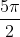radians/seconds.  How long will it take the wheel to make 10 full rotations?

1 second

8 seconds

24 seconds

20 seconds

8 seconds

Explanation:

We know that the angular velocity to complete a full rotation (rotate through an angle of) isradians/seconds.  The angle of 10 full rotations will be 10 rotations through the angle, so we multiply these two quantities.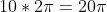And this will be our.  Now we must manipulate our angular velocity formula to solve for.Now we can plug in ourfor the 10 rotations and our angular velocity.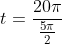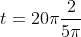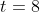And so it will take 8 seconds to make 10 rotations.

Example Question #8 : Angular Velocity

If it takes you 3 seconds to rotate through an angle with an angular velocity of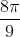, what is the measure of the angle you are rotating through?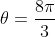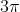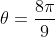Explanation:

We are able to use the angular velocity formula and solve for the unknown.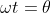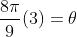(making it so we are solving for)